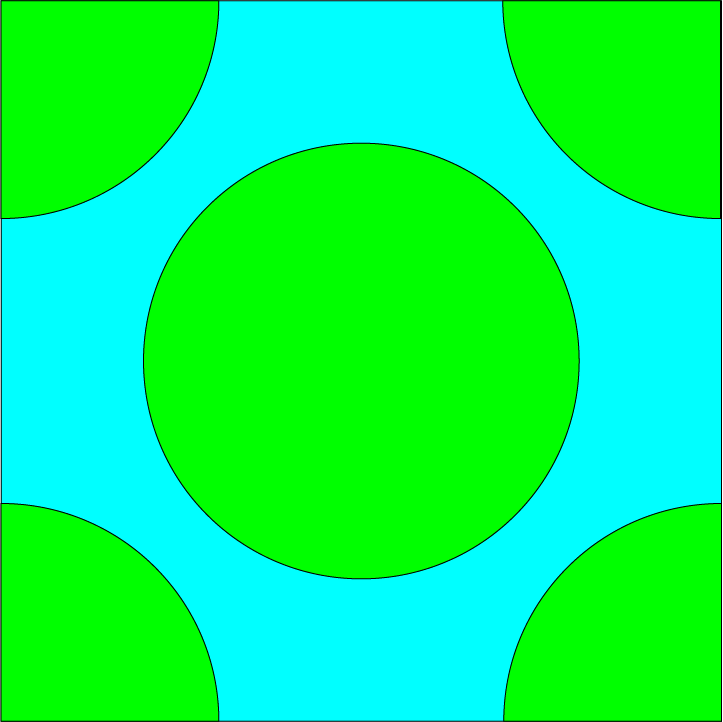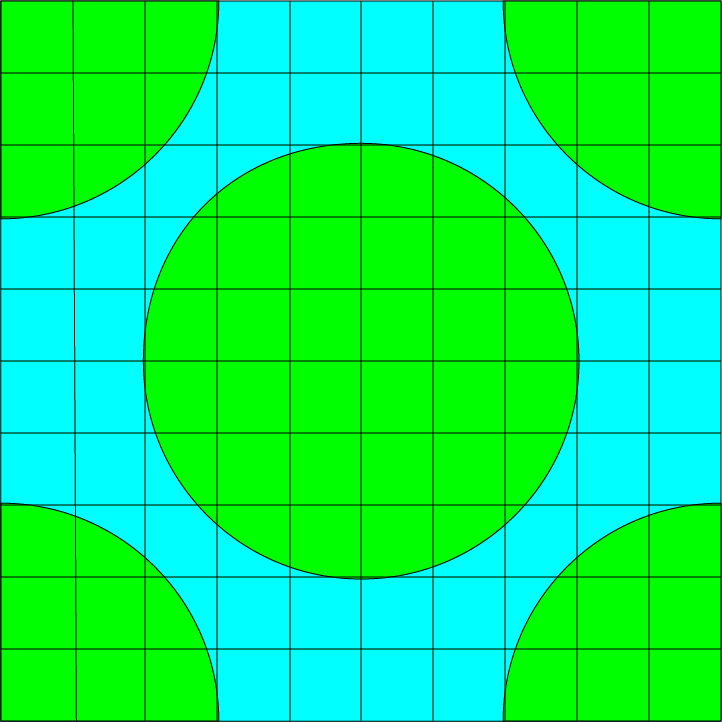# Solution: Which is Bigger?

## The Puzzle:

In the picture, is the area coloured green or the area coloured blue bigger?## The Solution:

Let's draw grid-lines over the puzzle so that it's easier to calculate the areas:The area of the square is $10 \times 10 = 100 \text{ square units}$.

The green areas are equivalent to the area of two circles of radius $3$. So,

$A(\text{green}) = 2 \times \pi \times 3^2 \approx 56.55 \text{ square units}$,
and
$A(\text{blue}) = 100 - 56.55 = 43.45 \text{ square units}$.
So the green area is bigger.

Note: If I'd made the green circles just a big smaller, the blue area would have been bigger. For example, if I made the radius $2.8$, the green area would have been approximately $47.16 \text{ square units}$, and the blue area would have been \(52.84 \text{ square units}), which is larger.

### Description

This series of puzzles are for Year 10 or higher students, these puzzles tests your skills and also train you with problem solving and thinking out of the box

### Audience

Year 10 students or higher

### Learning Objectives

Solving puzzles

Author: Subject Coach
You must be logged in as Student to ask a Question.